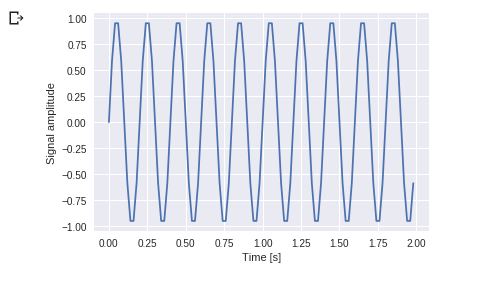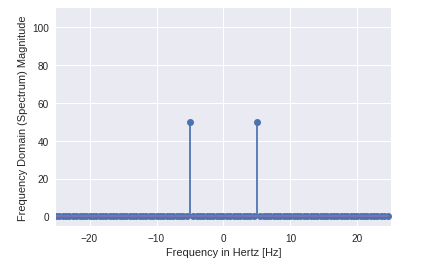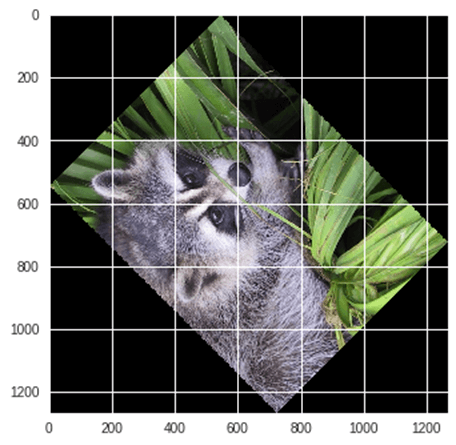﻿ Python 教程中的 SciPy：什么是 |库和函数示例MfgRobots >> 亿迅智能制造网 >  >> Industrial programming >> Python
Python 教程中的 SciPy：什么是 |库和函数示例

## Numpy VS SciPy

### 麻木：

• Numpy 是用 C 语言编写的，用于数学或数值计算。
• 它比其他 Python 库更快
• Numpy 是数据科学执行基本计算的最有用的库。
• Numpy 只包含数组数据类型，它执行最基本的操作，如排序、整形、索引等。

### SciPy：

• SciPy 内置于 NumPy 之上
• Python 中的 SciPy 模块是线性代数的全功能版本，而 Numpy 仅包含一些功能。
• 大多数新的数据科学功能在 Scipy 中可用，而不是在 Numpy 中。

## SciPy – 安装和环境设置

`Python3 -m pip install --user numpy scipy `

`sudo apt-get install python-scipy python-numpy`

`sudo 端口安装 py35-scipy py35-numpy`

`from scipy import special #same for other modulesimport numpy as np`

## 文件输入/输出包：

Scipy，I/O 包，具有​​广泛的功能，可以处理不同的文件格式，包括 Matlab、Arff、Wave、Matrix Market、IDL、NetCDF、TXT、CSV 和二进制格式。

` import numpy as np from scipy import io as sio array =np.ones((4, 4)) sio.savemat('example.mat', {'ar':array}) data =sio.loadmat(' example.mat', struct_as_record=True) data['ar']`

`array([[ 1., 1., 1., 1.], [ 1., 1., 1., 1.], [ 1., 1., 1., 1.], [ 1 ., 1., 1., 1.]])`

• 第 1 行和第 2 行： 使用 I/O 包和 Numpy 在 Python 中导入基本的 SciPy 库。
• 第 3 行 :创建 4 x 4 维数组
• 第 4 行 :在 example.mat 中存储数组 文件。
• 第 5 行：example.mat 获取数据 文件
• 第 6 行 :打印输出。

## 特殊功能包

• scipy.special 包中包含许多数学物理函数。
• SciPy 特殊函数包括立方根、指数、对数和指数、兰伯特、排列和组合、伽玛、贝塞尔、超几何、开尔文、β、抛物线柱面、相对误差指数等。
• 对于所有这些函数的一行描述，请在 Python 控制台中输入：
`help(scipy.special) 输出：NAME scipy.specialDESCRIPTION ==========================================特殊功能 (:mod:`scipy.special`) ==========================================.. module::scipy.special 下面的几乎所有函数都是通用函数，并遵循广播和自动数组循环规则。例外情况已注明。`

### 三次根函数：

Cubic Root 函数求值的立方根。

`scipy.special.cbrt(x)`

`from scipy.special import cbrt#用cbrt()函数求27&64的立方根cb =cbrt([27, 64])#打印cbprint(cb)的值`

### 指数函数：

`from scipy.special import exp10#define exp10 函数并在其exp =exp10([1,10])print(exp)中传递值`

### 排列组合：

SciPy 还提供了计算排列和组合的功能。

`from scipy.special import comb#find 5, 2 个值的组合使用 comb(N, k)com =comb(5, 2, exact =False, repeat=True)print(com)`

`scipy.special.perm(N,k)`

`from scipy.special import perm#find permutation of 5, 2 using perm (N, k) functionper =perm(5, 2, exact =True)print(per)`

### 对数和指数函数

Log Sum Exponential 计算和指数输入元素的对数。

`scipy.special.logsumexp(x) `

### 贝塞尔函数

`scipy.special.jn()`

## SciPy 的线性代数

• SciPy 的线性代数是 BLAS 和 ATLAS LAPACK 库的实现。
• 与 BLAS 和 LAPACK 相比，线性代数的性能非常快。
• 线性代数例程接受二维数组对象，输出也是二维数组。

`from scipy import linalgimport numpy as np#define square matrixtwo_d_array =np.array([ [4,5], [3,2] ])#pass values to det() functionlinalg.det( two_d_array )`

`scipy.linalg.inv()`

Scipy的逆矩阵计算任何方阵的逆。

`from scipy import linalgimport numpy as np#define square matrixtwo_d_array =np.array([ [4,5], [3,2] ])#pass value to function inv()linalg.inv( two_d_array )`

`数组( [[-0.28571429, 0.71428571], [ 0.42857143, -0.57142857]] )`

### 特征值和特征向量

scipy.linalg.eig()

• 线性代数中最常见的问题是特征值和特征向量，它们可以使用 eig() 轻松解决 功能。
• 现在让我们找到 (X ) 并对应一个二维方阵的特征向量。

`from scipy import linalgimport numpy as np#define 二维数组arr =np.array([[5,4],[6,3]])#pass value into functioneg_val, eg_vect =linalg.eig(arr)#get特征值print(eg_val)#get eigenvectorsprint(eg_vect)`

`[ 9.+0.j -1.+0.j] #eigenvalues [ [ 0.70710678 -0.5547002 ] #eigenvectors [ 0.70710678 0.83205029] ]`

## 离散傅立叶变换——scipy.fftpack

• DFT 是一种数学技术，用于将空间数据转换为频率数据。
• FFT（快速傅立叶变换）是一种计算 DFT 的算法
• FFT 应用于多维数组。
• 频率定义了特定时间段内信号或波长的数量。

`%matplotlib inlinefrom matplotlib import pyplot as pltimport numpy as np #Frequency 在 Hertzfre =5 方面 #Sample ratefre_samp =50t =np.linspace(0, 2, 2 * fre_samp, endpoint =False )a =np.sin( fre * 2 * np.pi * t)figure, axis =plt.subplots()axis.plot(t, a)axis.set_xlabel ('Time (s)')axis.set_ylabel ('信号幅度')plt.show ()``from scipy import fftpackA =fftpack.fft(a)frequency =fftpack.fftfreq(len(a)) * fre_sampfigure,axis =plt.subplots()axis.stem(frequency, np.abs(A))axis。 set_xlabel('Frequency in Hz')axis.set_ylabel('Frequency Spectrum Magnitude')axis.set_xlim(-fre_samp / 2, fre_samp/ 2)axis.set_ylim(-5, 110)plt.show()`• 您可以清楚地看到输出是一个一维数组。
• 包含复数值的输入为零，但两点除外。
• 在 DFT 示例中，我们将信号的幅度可视化。

## SciPy 中的优化和适配 – scipy.optimize

• 优化提供了一种有用的算法，用于最小化曲线拟合、多维或标量和根拟合。
• 标量函数为例 找到最小标量函数
`%matplotlib inlineimport matplotlib.pyplot as pltfrom scipy import optimizeimport numpy as npdef function(a):return a*2 + 20 * np.sin(a)plt.plot(a, function(a))plt.show( )#使用BFGS算法优化optimize.fmin_bfgs(function, 0) `• 在这个例子中，优化是在梯度下降算法的帮助下从初始点开始的
• 但可能的问题是局部最小值而不是全局最小值。如果我们没有找到全局最小值的邻居，那么我们需要应用全局优化并找到用作basinhopping()的全局最小值函数 它结合了本地优化器。

optimize.basinhopping(function, 0)

`fun:-23.241676238045315 minimum_optimization_result:fun:-23.241676238045315 hess_inv:array([[0.05023331]]) jac:array([4.76837158e] 消息成功终止：'07 nfev:15 nit:3 njev:5 status:0 success:True x:array([-1.67096375]) 消息：['请求的盆地跳跃迭代次数成功完成'] minimization_failures:0 nfev:1530 nit:100 njev:510 x :数组([-1.67096375])`

• 它为公平行为函数提供了最直接的最小化方法。
`import numpy as npfrom scipy.optimize import minimum#define function f(x)def f(x):return .4*(1 - x)**2 optimize.minimize(f, [2, - 1], method="Nelder-Mead")`

`final_simplex:(array([[ 1. , -1.27109375], [ 1. , -1.27118835], [ 1. , -1.27113762]]), array([0., 0., 0.])) fun ：0.0 消息：“优化已成功终止。” nfev:147 nit:69 状态:0 成功:True x:array([ 1., -1.27109375])`

## 使用 SciPy 进行图像处理 – scipy.ndimage

• scipy.ndimage 是 SciPy 的一个子模块，主要用于执行与图像相关的操作
• ndimage 表示“n”维图像。
• SciPy 图像处理提供几何变换（旋转、裁剪、翻转）、图像过滤（锐化和去噪）、显示图像、图像分割、分类和特征提取。
• MISC 包 SciPy 中包含可用于执行图像处理任务的预构建图像

`from scipy import miscfrom matplotlib import pyplot as pltimport numpy as np#get face image of panda from misc packagepanda =misc.face()#plot or show image of faceplt.imshow( panda )plt.show()``#Flip Down using scipy misc.face image flip_down =np.flipud(misc.face())plt.imshow(flip_down)plt.show()``from scipy import ndimage, miscfrom matplotlib import pyplot as pltpanda =misc.face()#scipy for image的旋转函数——图像旋转135度panda_rotate =ndimage.rotate(panda, 135)plt.imshow(panda_rotate)plt.show ()`### 与 Scipy 集成 - 数值集成

• 当我们对无法进行解析积分的任何函数进行积分时，我们需要转向数值积分
• SciPy 提供了将函数与数值积分相结合的功能。
• scipy.integrate 库具有单积分、双积分、三重、多次积分、高斯二次方、Romberg、梯形和辛普森规则。`from scipy import integration# take f(x) function as ff =lambda x :x**2#single integration with a =0 &b =1 integration =integr.quad(f, 0 , 1)print(integration )`

(0.33333333333333337, 3.700743415417189e-15)`from scipy import integrationimport numpy as np#import square root function from math libfrom math import sqrt# set fuction f(x)f =lambda x, y :64 *x*y# 第二个积分的下限p =lambda x :0# 第一积分上限 q =lambda y :sqrt(1 - 2*y**2)# perform double integrationintegration =integration.dblquad(f , 0 , 2/4, p, q)print(integration)`

(3.0, 9.657432734515774e-14)

## 总结

• SciPy（发音为“Sigh Pi”）是一个基于 Python 的开源库，可用于数学、科学计算、工程和技术计算。
• SciPy 包含各种子包，有助于解决与科学计算相关的最常见问题。
• SciPy 内置于 NumPy 之上

scipy.io
• 文件输入/输出
scipy.special
• 特殊功能
scipy.linalg
• 线性代数运算
scipy.interpolate
• 插值
scipy.optimize
• 优化与适配
scipy.stats
• 统计和随机数
scipy.integrate
• 数值积分
scipy.fftpack
• 快速傅立叶变换
scipy.signal
• 信号处理
scipy.ndimage
• 图像处理——

Python